4402.0 - Child Care, Australia, Jun 2005 Second Reissue
ARCHIVED ISSUE Released at 11:30 AM (CANBERRA TIME) 19/11/2008
 Page tools: .mffeedback,#pjs { display :none; } Enable Javascript to Print PagesPrint All Explanatory Notes Glossary Abbreviations Data Items List (Appendix) Data Cubes (I-Note) Data Quality (Technical Note) Standard Errors Data Source TECHNICAL NOTE DATA QUALITY ESTIMATION PROCEDURE 1 Estimates of children are derived using a ratio estimation procedure which ensures that estimates conform to an independently estimated state by age by sex distribution of children in the population, rather than to the state by age by sex distribution within the sample itself. Estimates of families conform to an independently estimated state by household composition distribution in the population, where household composition was defined by the number of adults and children within a household. RELIABILITY OF ESTIMATES 2 Since the estimates in this publication are based on information obtained from occupants of a sample of dwellings, they are subject to sampling variability. That is, they may differ from those estimates that would have been produced if all dwellings had been included in the survey. One measure of the likely difference is given by the standard error (SE), which indicates the extent to which an estimate might have varied by chance because only a sample of dwellings was included. There are about two chances in three (67%) that a sample estimate will differ by less than one SE from the number that would have been obtained if all dwellings had been included, and about 19 chances in 20 (95%) that the difference will be less than two SEs. Another measure of the likely difference is the relative standard error (RSE), which is obtained by expressing the SE as a percentage of the estimate. 3 Due to space limitations, it is impractical to print the SE of each estimate in the publication. Instead, a table of SEs is provided to enable readers to determine the SE for an estimate from the size of that estimate (see tables T1 and T2). There are separate SE tables for estimates of number of children and estimates of number of families. Each SE table is derived from a mathematical model, referred to as the 'SE model', which is created using the data collected in this survey. It should be noted that the SE model only gives an approximate value for the SE for any particular estimate, since there is some minor variation among SEs for different estimates of the same size. CALCULATION OF STANDARD ERRORS 4 An example of the calculation and the use of SEs in relation to estimates of children is as follows. Calculation of SEs relating to estimates of families use table T2 instead of table T1. Table 1 shows that the estimated number of children aged 3 who used formal care only is 81,100. Since this estimate is between 50,000 and 100,000, table T1 shows that the SE for Australia will lie between 5,050 and 7,050 and can be approximated by interpolation using the following general formula: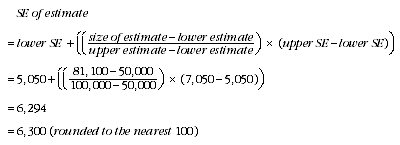5 Therefore, there are about two chances in three that the value that would have been produced if all dwellings had been included in the survey will fall within the range 74,800 to 87,400 and about 19 chances in 20 that the value will fall within the range 68,500 to 93,700. This example is illustrated in the diagram below.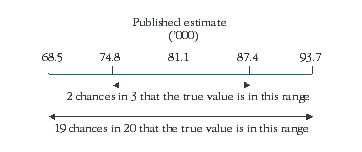6 In general, the size of the SE increases as the size of the estimate increases. Conversely, the RSE decreases as the size of the estimate increases. Very small estimates are thus subject to such high RSEs so that their value for most practical purposes is unreliable. In the tables in this publication, only estimates with RSEs of 25% or less are considered reliable for most purposes. Estimates with RSEs greater than 25% but less than or equal to 50% are preceded by an asterisk (e.g. *3.4) to indicate they are subject to high SEs and should be used with caution. Estimates with RSEs of greater than 50%, preceded by a double asterisk (e.g. **0.3), are considered too unreliable for general use and should only be used to aggregate with other estimates to provide derived estimates with RSEs of 25% or less. PROPORTIONS AND PERCENTAGES 7 Proportions and percentages formed from the ratio of two estimates are also subject to sampling errors. The size of the error depends on the accuracy of both the numerator and the denominator. A formula to approximate the RSE of a proportion is given below. This formula is only valid when x is a subset of y.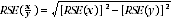8 For example, in table 1 the estimate for the total number of children aged 3 who used child care (formal and/or informal) is 175,300. The estimated number of children aged 3 who used formal care only is 81,100, so the proportion of children aged 3 who used formal care only out of those children who used child care is 81,100/175,300 or 46.3%. The SE of the total number of children aged 3 who used child care may be calculated by interpolation as 9,100 (rounded to the nearest 100). To convert this to an RSE we express the SE as a percentage of the estimate, which gives 9,100/175,300 = 5.2%. The SE for the number of children aged 3 who used formal care only was calculated above as 6,300, which converted to an RSE is 6,300/81,100 = 7.8%. Applying the above formula, the RSE of the proportion is: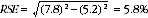giving a SE for the proportion (46.3%) of 2.7 percentage points (=46.3*5.8/100). 9 Therefore, there are about two chances in three that the proportion of children aged 3 who used formal care only out of those who used child care is between 43.6% and 49.0% and 19 chances in 20 that the proportion is within the range 40.9% to 51.7%. DIFFERENCES 10 Published estimates may also be used to calculate the difference between two survey estimates (of numbers or percentages). Such an estimate is subject to sampling error. The sampling error of the difference between two estimates depends on their SEs and the relationship (correlation) between them. An approximate SE of the difference between two estimates (x-y) may be calculated by the following formula: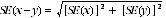11 While this formula will only be exact for differences between separate and uncorrelated characteristics or subpopulations, it is expected to provide a good approximation for all differences likely to be of interest in this publication. Document Selection These documents will be presented in a new window.